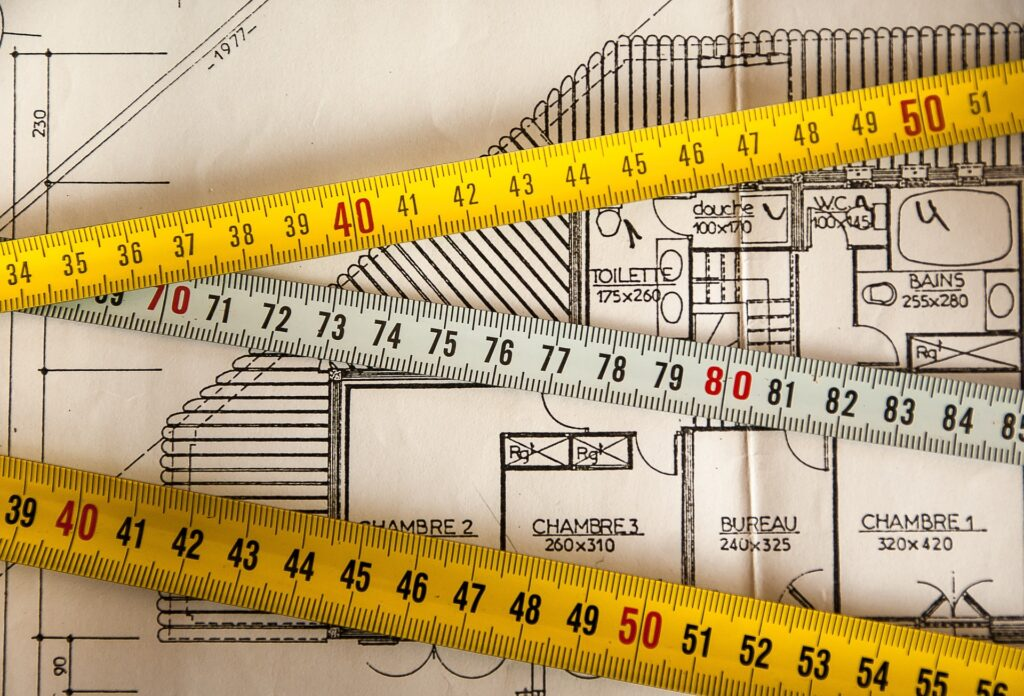## Your Imagination is Our CreationArea Conversion Calculator

# Area Conversion Calculator

Result:

When it comes to measuring land or property, there are several units that we commonly use – square meters (sqm), square feet (sqft), acres, and square yards (sqyd). Each unit measures the area of a space, or how much ground it covers.

Here are the formulas used to convert between these units:

1. Square meters to square feet: Multiply the number of square meters by 10.7639.
• This means that for every square meter, there are 10.7639 square feet. So if you have 10 square meters, you would multiply 10 by 10.7639 to get 107.639 square feet.
1. Square feet to square meters: Divide the number of square feet by 10.7639.
• This is the opposite of the first formula. If you have 100 square feet, you would divide 100 by 10.7639 to get 9.2903 square meters.
1. Square meters to acres: Divide the number of square meters by 4046.86.
• An acre is a larger unit of area that is commonly used to measure land. If you have 1000 square meters, you would divide 1000 by 4046.86 to get 0.2471 acres.
1. Acres to square meters: Multiply the number of acres by 4046.86.
• This is the opposite of the third formula. If you have 5 acres, you would multiply 5 by 4046.86 to get 20234.3 square meters.
1. Square meters to square yards: Multiply the number of square meters by 1.19599.
• Square yards are another unit of area that is commonly used. If you have 50 square meters, you would multiply 50 by 1.19599 to get 59.7995 square yards.
1. Square yards to square meters: Divide the number of square yards by 1.19599.
• This is the opposite of the fifth formula. If you have 200 square yards, you would divide 200 by 1.19599 to get 167.2301 square meters.

We hope that explains the conversions in a clear and simple way!

Length Conversion Calculator

# Length Conversion Calculator

The calculator allows you to convert between three different units of length: feet, inches, and meters. Here’s a brief explanation of each of these units:

• Feet: A foot is a unit of length equal to 12 inches. It’s commonly used to measure height or distance in the US.
• Inches: An inch is a unit of length equal to 1/12 of a foot. It’s commonly used to measure small distances or lengths.
• Meters: A meter is a unit of length in the metric system. It’s defined as the distance traveled by light in a vacuum in 1/299,792,458 of a second.

#### To convert between these units, we need to use some simple formulas. Here are the formulas used in the calculator:

Feet to Inches: To convert feet to inches, we multiply the number of feet by 12.

• For example, if we want to convert 5 feet to inches, we multiply 5 by 12 to get 60 inches.

Feet to Meters: To convert feet to meters, we multiply the number of feet by 0.3048.

• For example, if we want to convert 5 feet to meters, we multiply 5 by 0.3048 to get 1.524 meters.

Inches to Feet: To convert inches to feet, we divide the number of inches by 12.

• For example, if we want to convert 60 inches to feet, we divide 60 by 12 to get 5 feet.

Inches to Meters: To convert inches to meters, we multiply the number of inches by 0.0254.

• For example, if we want to convert 60 inches to meters, we multiply 60 by 0.0254 to get 1.524 meters.

Meters to Feet: To convert meters to feet, we divide the number of meters by 0.3048.

• For example, if we want to convert 1.524 meters to feet, we divide 1.524 by 0.3048 to get 5 feet.

Meters to Inches: To convert meters to inches, we multiply the number of meters by 39.37.

• For example, if we want to convert 1.524 meters to inches, we multiply 1.524 by 39.37 to get 60 inches.

We hope this helps you understand the formulas used in the length conversion calculator!